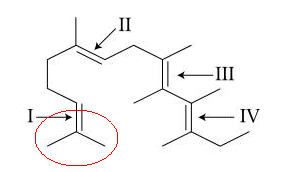Filter By

√3e

Option d

## Crack CUET with india's "Best Teachers"

• HD Video Lectures
• Unlimited Mock Tests
• Faculty Support#### Letbe such that the equation,has a repeated root, which is also a root of the equation,. Ifis the other root of this equation, thenis equal to: Option: 1Option: 2Option: 3Option: 4Nature of Roots -

Let the quadratic equation is ax2 + bx + c = 0

D is the discriminant of the equation.

iii) if roots D = 0, then roots will be real and equal, then-

ax2 – 2bx + 5 = 0 having equal roots orandPutin the second equationCorrect Option 2

#### The  number of real roots of the equation,is :    Option: 1Option: 2 4 Option: 3 1 Option: 4 2

Transcendental function -

Transcendental functions:  the functions which are not algebraic are called transcendental functions. Exponential, logarithmic, trigonometric and inverse trigonometric functions are transcendental functions.

Exponential Function: function f(x) such thatis known as an exponential function.Logarithmic function:  function f(x) such thatis called logarithmic functionIf a > 1                                                                               If a < 1

Properties of Logarithmic Function-

The root of the quadratic equation is given by the formula:Where D is called the discriminant of the quadratic equation, given by,

-

LetNow the equationLetOnly positive value possible sox=0 is the only solution.

## Crack NEET with "AI Coach"

• HD Video Lectures
• Unlimited Mock Tests
• Faculty Support#### At 300 K and 1 atm, 15 mL of a gaseous hydrocarbon requires 375 mL air containing 20% O2 by volume for complete combustion.  After combustion, the gases occupy 330 mL.  Assuming that the water formed is in liquid form and the volumes were measured at the same temperature and pressure, the formula of the hydrocarbon is : Option: 1  C4H8   Option: 2  C4H10 Option: 3  C3H6 Option: 4  C3H8

Volume of N in air = 375 × 0.8 = 300 ml

Volume of O2 in air = 375 × 0.2 = 75 ml

15ml

0                         0                            15x                 -

After combustion total volume

330 = 300 + 15x

x = 2

Volume of O2 used

y = 12

So hydrocarbon is = C2H12

None of the options matches it therefore it is a BONUS.

----------------------------------------------------------------------

Alternatively  Solution

15ml

0                         0                            15x                 -

Volume of O2 used

If further information (i.e., 330 ml) is neglected, option (C3H8 ) only satisfy the above equation.

#### An ideal gas undergoes a quasi static, reversible process in which its molar heat capacity C remains constant.  If during  this process the relation of pressure P and volume V is given by PVn=constant,  then n is given by (Here CP and CV are molar specific heat at constant pressure and constant volume, respectively) Option: 1   Option: 2   Option: 3  Option: 4

For a polytropic preocess## Crack JEE Main with "AI Coach"

• HD Video Lectures
• Unlimited Mock Tests
• Faculty Support#### A point particle of mass m, moves along the uniformly rough track PQR as shown in the figure.  The coefficient of friction, between the particle and the rough track equals µ.  The particle is released, from rest, from the point P and it comes to rest at a point R.  The energies, lost by the ball, over the parts, PQ and QR, of the track, are equal to each other, and no energy is lost when particle changes direction from PQ to QR. The values of the coefficient of friction µ and the distance x(=QR), are, respectively close to :Option: 1  0.2 and 6.5 m   Option: 3  0.2 and 3.5 m   Option: 4   0.29 and 6.5 m

Work done by friction at QR = μmgx

In triangle, sin 30° = 1/2 = 2/PQ

PQ = 4 m

Work done by friction at PQ = μmg × cos 30° × 4 = μmg × √3/2 × 4 = 2√3μmg

Since work done by friction on parts PQ and QR are equal,

μmgx = 2√3μmg

x = 2√3 ≅ 3.5 m

Applying work energy theorem from P to R

decrease in P.E.=P.E.= loss of energy due to friction in PQPQ and QR

where h=2(given)

#### In the following structure, the double bonds are marked as I, II, III and IVGeometrical isomerism is not possible at site (s) : Option: 1  III Option: 2  I Option: 3  I and II Option: 4  III and IV

Geometrical isomerism is not possible at Site I as two identical methyl groups are attached to the same carbon bearing the double bond.Hence, the answer is Option (2)

## Crack CUET with india's "Best Teachers"

• HD Video Lectures
• Unlimited Mock Tests
• Faculty Support#### Which one of the following is an oxide ? Option: 1  KO2 Option: 2  BaO2 Option: 3  SiO2 Option: 4 CsO2SiO2  - oxide

KO???2? , CsO????2  -  superoxides

BaO????2 -  peroxide

#### The following reaction occurs in the Blast Furnace where iron ore is reduced to iron metal : Fe2O3(s)+3 CO(g) 2 Fe(l)+3 CO2(g) Using the Le Chatelier’s principle, predict which one of the following will not disturb the equilibrium ? Option: 1  Removal of CO Option: 2  Removal of CO2 Option: 3 Addition of CO2 Option: 4 Addition of Fe2O3According to Le Chatelier's principle change in concentration by changing the amount of reactant or product affect the equilibrium. However, the addition of solid reactant won't affect the concentration.

Therefore, addition of solid Fe2O???3 will not disturb the equilibrium.